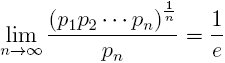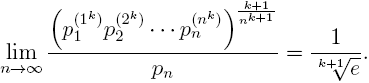# On a limit where appear prime numbers

Rafael Jakimczuk
Notes on Number Theory and Discrete Mathematics
Print ISSN 1310–5132, Online ISSN 2367–8275
Volume 22, 2016, Number 1, Pages 1—4

## Details

### Authors and affiliations

Rafael JakimczukDivisión Matemática, Universidad Nacional de Luján
Buenos Aires, Argentina

### Abstract

Let pn be the n-th prime number. The following limit is well-knownLet k be a fixed but arbitrary nonnegative integer. In this note we prove the more general limit### Keywords

• Prime numbers
• The e number
• Limits

• 11A99
• 11B99

### References

1. Jakimczuk, R. (2007) The Ratio between the Average Factor in a Product and the Last Factor, Mathematical Sciences: Quarterly Journal, 1, 53–62.
2. Jakimczuk, R. (2013) Sums of perfect powers, International Journal of Contemporary Mathematical Sciences, 8, 61–67.
3. Jakimczuk, R. (2012) Asymptotic formulae for the n-th perfect power, Journal of Integer Sequences, 15, Article 12.5.5.
4. Sándor J., & Verroken A. (2011) On a limit involving the product of prime numbers, Notes on Number Theory and Discrete Mathematics, 17(2), 1–3.
5. Sándor J. (2012) On certain bounds and limits for prime numbers, Notes on Number Theory and Discrete Mathematics, 18(1), 1–5.

## Cite this paper

APA

Jakimczuk, R. (2016). On a limit where appear prime numbers. Notes on Number Theory and Discrete Mathematics, 22(1), 1-4.

Chicago

Jakimczuk, Rafael. “On a limit where appear prime numbers.” Notes on Number Theory and Discrete Mathematics 22, no. 1 (2016): 1-4.

MLA

Jakimczuk, Rafael. “On a limit where appear prime numbers.” Notes on Number Theory and Discrete Mathematics 22.1 (2016): 1-4. Print.